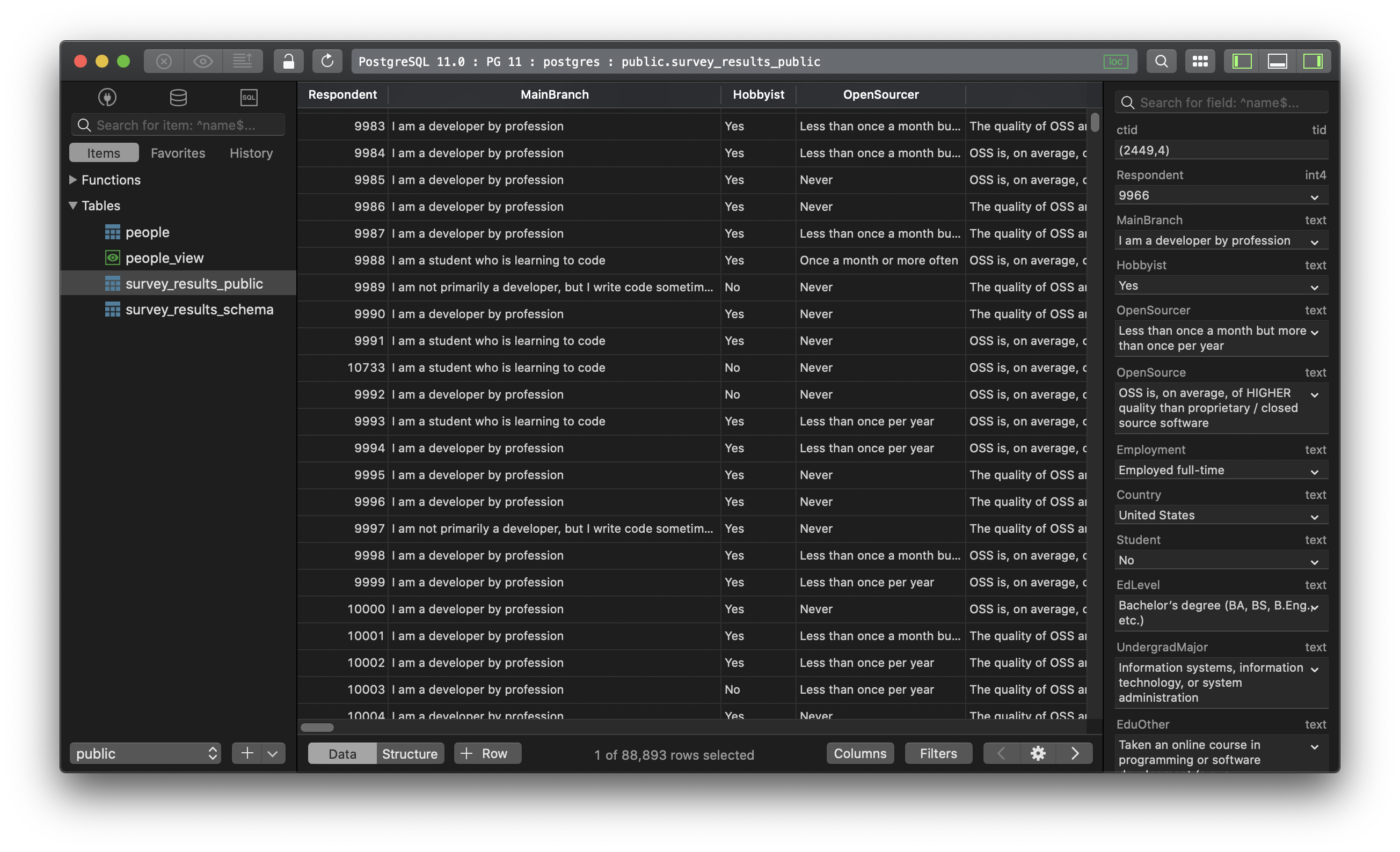In PostgreSQL, the `random()` function does the job of to generating a random number from 0 to 1. In this post, we are going to use this `random()` function to generate a random number within a specified range.

To generate a random number between 0 and 1, execute this command:

``````SELECT random() AS a_random_no;
``````
• To return a random number from a bigger range, let’s say from 0 to 100:
``````SELECT random() * 100 AS a_random_no;
``````
• If you want the output to be an integer, use the floor() function:
``````SELECT floor(random() * 100) AS a_random_no;
``````

To be more general, you want to generate a random number in between two particular numbers a and b, where a is smaller than b, run this command:

``````SELECT floor(random() * (b - a + 1)) + a AS a_random_no;
``````

For example, select a random number from between 5 and 105:

``````SELECT floor(random() * (105 - 5 + 1)) + 5 AS random_no;
``````

Need a good GUI tool for PostgreSQL, or MySQL and many other databases? Check out TablePlus. It’s native, beautiful, and available for free.